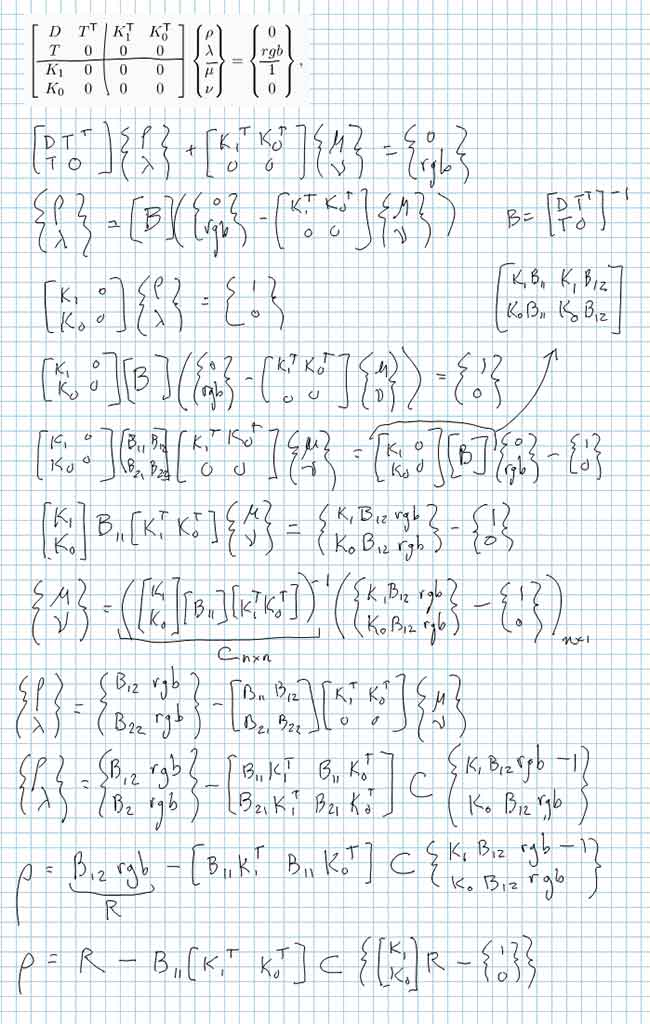# Generating Reflectance Curves from sRGB Triplets (Page 7)

#### Iterative Least Slope Squared (ILSS) Method

For completeness, I thought it would be a good idea to add one more algorithm. Recall the ILLSS method modifies the LLSS method to cap reflectances >1. Similarly, the ILSS method will modify the LSS method to cap values both >1 and <0. The ILSS may reduce computational effort in comparison to the ILLSS method since the inner loop of the ILLSS method requires an iterative Newton’s method solution, whereas there would be no inner loop needed with the ILSS method; it is simply the solution of a linear system of equations. Here is the ILSS formulation: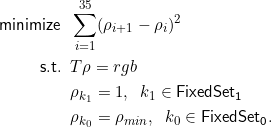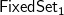is the set of reflectance indices that are constrained to equal 1, and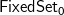is the set of indices that are constrained to equal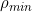(the smallest allowable reflectance, typically 0.00001). Initially, both fixed sets are the empty set. Each time the optimization is repeated, the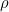values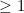have their indices added toand those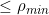have their indices added to.

We define two matrices that summarize the fixed sets, for example: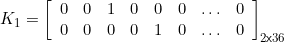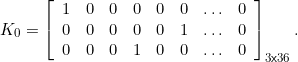This example indicates that there are two reflectance values being constrained to equal 1 (because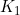has two rows), and the third and fifth reflectance values are the particular ones being constrained. There are three reflectance values being constrained to(because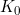has three rows), and the first, sixth, and fourth are the particular ones being constrained. The order in which the rows appear in these matrices is not important.

At each iteration of the ILSS method, this linear system is solved: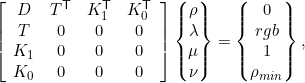where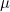and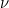are Lagrange multipliers associated with theandsets, respectively.

This system can be solved for, yielding the expression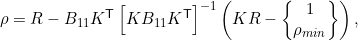where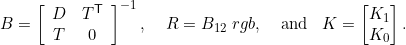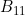and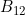are the upper-left 36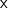36 and upper-right 363 parts of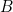, respectively. They can be computed ahead of time. Note that only an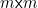matrix needs to be inverted at each iteration, where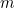is the number ofvalues being held fixed, typically zero or a small number. Whenis zero, the ILSS method simplifies to the LSS method. Here is a Matlab program that performs the ILSS (Iterative Least Slope Squared) method. It also works in Octave. Matlab code can be dense and difficult to read, so I’ve prepared a line-by-line commentary for understanding the code.

Derivation
Here are my notes on deriving the expression for: